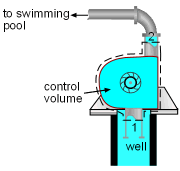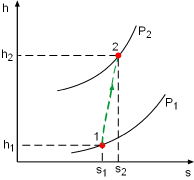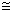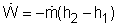Ch 6. Entropy Multimedia Engineering Thermodynamics Entropy Tds Relations EntropyChange IsentropicProcess IsentropicEfficiency EntropyBalance (1) EntropyBalance (2) ReversibleWork
 Chapter 1. Basics 2. Pure Substances 3. First Law 4. Energy Analysis 5. Second Law 6. Entropy 7. Exergy Analysis 8. Gas Power Cyc 9. Brayton Cycle 10. Rankine Cycle Appendix Basic Math Units Thermo Tables Search eBooks Dynamics Fluids Math Mechanics Statics Thermodynamics Author(s): Meirong Huang Kurt Gramoll ©Kurt GramollTHERMODYNAMICS - CASE STUDY SOLUTION A used pump must match the motor in Mr. Williams' swimming pool. The power required to run the pump needs to be determined. Assumptions: Model the pump as an adiabatic one. The flow process in the pump is steady. The kinetic and potential energies associated with water flowing through the pump are negligible. The pump operates at ambient temperature.The Control VolumeThe Pumping Process on the h-s Diagram Take the pump as a control volume, shown on the left. Denote the inlet by subscript 1 and exit by subscript 2. The definition of isentropic efficiency of pump is:       η Pv(P2 - P1)/(h2 - h1) It is assumed that the pump operates at ambient temperature. Hence the specific volume of water is       v = 0.001 m3/kg The pump increases the pressure of the water up to 300 kPa, which means the pressure difference of water between the exit and inlet is 300 kPa. That is       P2 - P1 = 300 kPa Substituting the given efficiency, which is 85%, and the pressure difference above to the definition of isentropic efficiency gives,       h2 - h1 = v(P2 - P1)/η P                  = 0.001(300,000)/0.85 = 352.9 J/kg With the assumptions that the pump is adiabatic and the kinetic and potential energies are negligible, the energy balance of the pump reduces toThe mass flow rate is given as 20 kg/min. Substituting the enthalpy difference and the mass flow rate to the energy balance yields,= -20/60(352.9) = -117.6 W The negative sign means the pump needs power input.